Space, shape and measurement: Solve problems by constructing and interpreting geometrical models

# Unit 1: Theorem of Pythagoras

Natashia Bearam-Edmunds### Unit outcomes: Unit 1: Transformation geometry

By the end of this unit you will be able to:

• Identify different types of triangles.
• Investigate the relationship between the sides of a right-angled triangle to develop the Theorem of Pythagoras.
• Use the Theorem of Pythagoras to find missing lengths in right angled triangles.

## What you should know

Before you start this unit, make sure you can:

• Identify different types of polygons. To revise the properties of polygons refer back to Subject outcome 3.1 Unit 1.
• Solve algebraic equations. You can revise how to solve algebraic equations and inequalities in Subject outcome 2.3.

## Introduction

Pythagoras was a Greek mathematician who developed one of the most famous theorems, which relates the sides of a right-angled triangle, called Pythagoras’ Theorem. Before we go into detail about Pythagoras’ Theorem, let’s recap the basics you need to know about triangles.

A triangle is a three-sided polygon. Triangles can be classified by their sides: equilateral, isosceles and scalene. Triangles can also be classified according to angles: acute-angled, right-angled and obtuse-angled. The different types of triangles are shown in Figure 1.

Different combinations of these properties are also possible. For example, you can get an obtuse isosceles triangle and a right-angled isosceles triangle. Try to draw these yourself. Remember that the sum of all the angles in any triangle will always be equal to $\scriptsize {{180}^{{}^\circ }}$.

## Investigating the sides of a right-angled triangle

As we have noted, a right-angled triangle has one $\scriptsize {{90}^{{}^\circ }}$ angle. The longest side of the right-angled triangle is found opposite the right-angle and is called the hypotenuse (pronounced ‘high – pot – eh – news’).

We use the notation △ABC to refer to a triangle with vertices labelled A, B and C in that order.### Activity 1.1: Investigate squares on the sides of a right-angled triangle

Time required: 15 minutes

What you need:

• a pen and paper

What to do:

Below is a right-angled triangle with squares on each of its sides.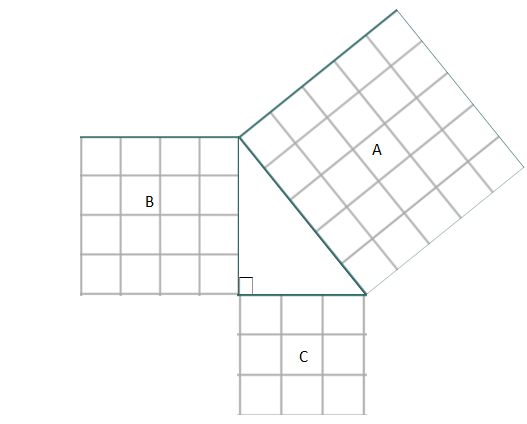1. Write down the areas of:
1. Square A
2. Square B
3. Square C
2. Add the area of square B and C. What do you get?
3. What do you notice about the areas of B plus C compared to A?
4. What is the length of the hypotenuse?
5. What is the length of each of the shorter sides of the triangle?
6. Wite an equation that relates the sum of the shorter sides of the triangle to the hypotenuse.

What did you find?

1. By counting you will get the following:
1. The area of $\scriptsize A=25$ square units.
2. The area of $\scriptsize \text{B}=16$ square units.
3. The area of $\scriptsize \text{C}=9$ square units.
2. $\scriptsize \text{Area of square B}+\text{Area of square C}=16+9=25$.
3. The areas of the sum of squares B and C is the same as the area of square A.
4. The hypotenuse measures $\scriptsize 5$ units.
5. The other two sides measure $\scriptsize 4$ and $\scriptsize 3$units.
6. $\scriptsize {{4}^{2}}+{{3}^{2}}={{5}^{{^{2}}}}$.

In Activity 1.1, you have discovered Pythagoras’ Theorem for right-angled triangles.

The Theorem of Pythagoras

In a right-angled triangle, a square formed on the hypotenuse will have the same area as the sum of the area of the two squares formed on the other sides of the triangle. Another way of saying this is that the square of the hypotenuse is equal to the sum of the squares of the other two sides.If △ABC is right-angled at angle B then $\scriptsize \text{A}{{\text{C}}^{2}}=\text{A}{{\text{B}}^{2}}+\text{B}{{\text{C}}^{2}}$.

Conversely, if $\scriptsize \text{A}{{\text{C}}^{2}}=\text{A}{{\text{B}}^{2}}+\text{B}{{\text{C}}^{2}}$ then △ABC is right-angled with $\scriptsize \hat{\text{B}}={{90}^{\circ }}$.

### Note

Remember that the Theorem of Pythagoras can only be used in a right-angled triangle.

When you have an internet connection you can view a demonstration of Pythagoras’ Theorem by watching the Pythagorean theorem water demo (Duration: 0.44).### Example 1.1

Use Pythagoras’ Theorem to determine whether these triangles are right-angled.

1. .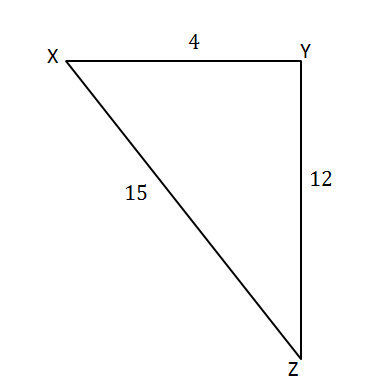2. .Solutions

1. If the triangle is right-angled the sum of the square of the two shorter sides will be equal to the square of the longest side.
$\scriptsize \begin{array}{l}\text{X}{{\text{Y}}^{2}}+\text{Y}{{\text{Z}}^{2}}&={{(4)}^{2}}+{{(12)}^{2}}\\&=16+144\\&=160\end{array}$
$\scriptsize \begin{array}{l}\text{X}{{\text{Z}}^{2}}={{(15)}^{2}}\\=225\\\therefore \text{X}{{\text{Z}}^{2}}\ne \text{X}{{\text{Y}}^{2}}+\text{Y}{{\text{Z}}^{2}}\end{array}$
Therefore, △XYZ is not right-angled since the sum of the square of the two shorter sides is not equal to the square of the longest side.
2. .
$\scriptsize \begin{array}{l}\text{L}{{\text{M}}^{2}}+\text{K}{{\text{M}}^{2}}&={{(20)}^{2}}+{{(21)}^{2}}\\&=841\end{array}$
Now, check KL.
$\scriptsize \begin{array}{l}\text{K}{{\text{L}}^{2}}&={{(29)}^{2}}\\&=841\end{array}$
$\scriptsize \therefore \text{L}{{\text{M}}^{2}}+\text{K}{{\text{M}}^{2}}=\text{K}{{\text{L}}^{2}}$
Therefore, △KLM is right-angled at angle M by the converse of Pythagoras.### Exercise 1.1

1. A triangle’s side lengths are $\scriptsize 8\text{ mm}$, $\scriptsize 15\text{ mm}$ and $\scriptsize \text{17 mm}$. Prove that it is a right-angled triangle.
2. Is a triangle with sides $\scriptsize 12\text{ cm}$, $\scriptsize 16\text{ cm}$and $\scriptsize \text{20 cm}$right-angled?

The full solutions are at the end of the unit.

### Did you know?

Did you know there are different ways to prove the Theorem of Pythagoras? Watch the video called “How many ways are there to prove the Pythagorean theorem?” to see these proofs.

How many ways are there to prove the Pythagorean theorem? (Duration: 05.16)## Finding missing sides

You can use the Theorem of Pythagoras to find the lengths of missing sides if you know that a triangle is right-angled.### Example 1.2

Calculate the length of the hypotenuse if the lengths of the other two sides are $\scriptsize 6$ units and $\scriptsize 8$ units.

Solution

Since we are told that the triangle has a hypotenuse this means the triangle is right-angled.

The sum of the square of the shorter sides is:

$\scriptsize \begin{array}{l}{{6}^{2}}+{{8}^{2}}&=36+64\\&=100\end{array}$

To find the length of the hypotenuse we need to take the square root of the sum of the squares of the shorter sides.

$\scriptsize \begin{array}{l}\text{hypotenus}{{\text{e}}^{2}}&=100\\ \therefore \text{hypotenuse}&=10\quad & \text{We only take the positive square root since distance is}\\&& \text{not negative}\text{.}\end{array}$

Sometimes the square root of a number is not a whole number or a rational number. In these cases, you can leave the answer under the square root sign. Remember, this is leaving your answer in surd form. Refer back to Unit 5 of Subject outcome 1.2 to revise surds.### Exercise 1.2

1. Find the length of the missing side in each of the triangles below. Leave the answers in surd form where applicable.
1. .2. .2. A rectangle has sides with lengths $\scriptsize 36\text{ mm}$ and $\scriptsize 77\text{ mm}$. Find the length of the rectangle’s diagonal.The full solutions are at the end of the unit.

## Summary

In this unit you have learnt the following:

• How to classify triangles according to their sides and angles.
• What the Theorem of Pythagoras states about the relationship between the sides of a triangle.
• How to prove a triangle is right-angled using the Theorem of Pythagoras.
• How to find missing sides using the Theorem of Pythagoras.

# Unit 1: Assessment

#### Suggested time to complete: 20 minutes

1. In the diagram below, distance JK and distance KL are $\scriptsize ~25$ metres while distance MK is $\scriptsize 20$ metres. Distance JM is equal to distance ML. Calculate the perimeter of the figure.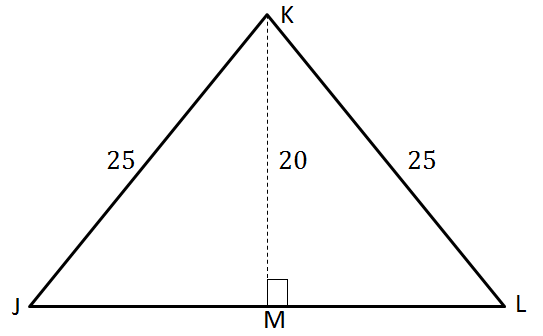2. An insect is observed walking around a rectangular garden. The distance from A to B is $\scriptsize ~12$ metres and the distance from A to C is $\scriptsize ~15$ metres.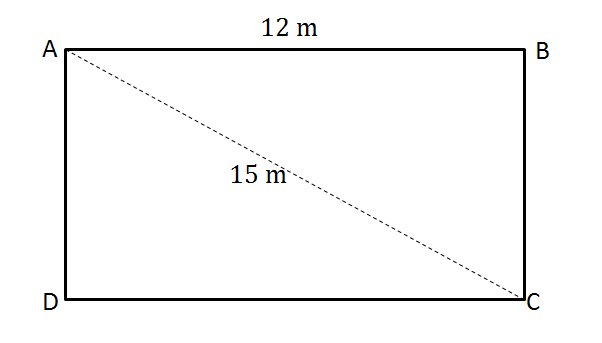Calculate the total distance that the insect walked.
3. An ambulance leaves the hospital parking (x) to travel to an emergency (y). The path and distance of the journey are shown by the sketch below.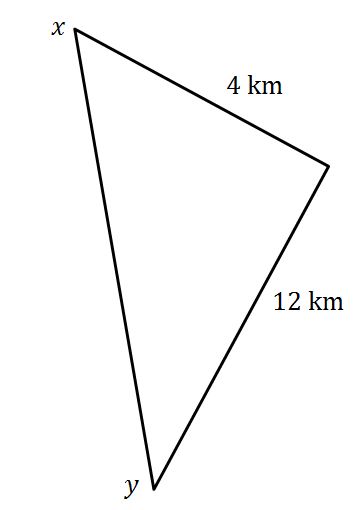Calculate the straight line distance between points x and y.

The full solutions are at the end of the unit.

# Unit 1: Solutions

### Exercise 1.1

1. .
$\scriptsize \begin{array}{l}{{(17\text{ mm})}^{2}}&=289\text{ m}{{\text{m}}^{2}}\\\text{And}\\{{(8\text{ mm})}^{2}}+{{(15\text{ mm})}^{2}}&=64\text{ m}{{\text{m}}^{2}}+225\text{ m}{{\text{m}}^{2}}\\&=289\text{ m}{{\text{m}}^{2}}\end{array}$
Therefore, the triangle is right angled by the converse of Pythagoras.
2. .
$\scriptsize \begin{array}{l}{{(20\text{ mm})}^{2}}&=400\text{ m}{{\text{m}}^{2}}\\\text{And}\\{{(16\text{ mm})}^{2}}+{{(12\text{ mm})}^{2}}&=256\text{ m}{{\text{m}}^{2}}+144\text{ m}{{\text{m}}^{2}}\\&=400\text{ m}{{\text{m}}^{2}}\end{array}$
Therefore, the triangle is right angled by the converse of Pythagoras.

Back to Exercise 1.1

Exercise 1.2

1. .
1. .
$\scriptsize \begin{array}{l}\text{K}{{\text{L}}^{2}}&=\text{K}{{\text{M}}^{2}}+\text{L}{{\text{M}}^{2}}\text{ (Pythagoras)}\\\therefore \text{L}{{\text{M}}^{2}}&=\text{K}{{\text{L}}^{2}}-\text{K}{{\text{M}}^{2}}\\&={{(17)}^{2}}-{{(15)}^{2}}\\&=289-225\\&=64\\\therefore \text{LM} &=\sqrt{{64}}\\&=8\end{array}$
2. .
$\scriptsize \begin{array}{l}\text{P}{{\text{Q}}^{2}}&=\text{Q}{{\text{R}}^{2}}+\text{P}{{\text{R}}^{2}}\text{ (Pythagoras)}\\&={{(55)}^{2}}\text{+(48}{{\text{)}}^{2}}\\&=5329\\\therefore \text{PQ}&=\sqrt{{5329}}\\&=73\end{array}$
2. A rectangle has interior angles each equal to $\scriptsize {{90}^{\circ }}$ so we can use the Theorem of Pythagoras to find the length of the diagonal.
$\scriptsize \begin{array}{l}{{(\text{Diagonal})}^{2}}&={{(36)}^{2}}+{{(77)}^{2}}\text{ (Pythagoras)}\\ &=7225\\\therefore \text{Diagonal}&=\sqrt{{7225}}\\&=85\text{ mm}\end{array}$

Back to Exercise 1.2

### Unit 1: Assessment

1. To calculate the perimeter of the figure we need to first find the length of ML.$\scriptsize \begin{array}{l}\text{In }\Delta \text{KML}\\\text{K}{{\text{M}}^{2}}+\text{M}{{\text{L}}^{2}} & =\text{K}{{\text{L}}^{2}}\text{ (Pythagoras)}\\\text{M}{{\text{L}}^{2}} & =\text{K}{{\text{L}}^{2}}-\text{K}{{\text{M}}^{2}}\\ & ={{(25)}^{2}}-{{(20)}^{2}}\\ & =225\\\therefore \text{ML} & =\sqrt{{225}}\\ & =15\\\text{JM} & =\text{ML}\\ & =15\end{array}$
$\scriptsize \begin{array}{l}\text{Perimeter} & =\text{JK}+\text{KL}+\text{JL}\\ & =25+25+(15+15)\\ & =80\end{array}$
2. To find the distance the insect walked we must find the perimeter of the rectangular garden.
.
$\scriptsize \begin{array}{l}\text{AB}=\text{DC}=12\text{ m}\quad \text{(opposite sides of a rectangle are equal)}\\\text{BC}=\text{AD}\end{array}$
.
To find $\scriptsize \text{BC}$, use Pythagoras in $\scriptsize \Delta \text{ABC}$ since $\scriptsize \hat{\text{B}}=90{}^\circ$.
$\scriptsize \begin{array}{l}\text{A}{{\text{B}}^{2}}+\text{B}{{\text{C}}^{2}} & =\text{A}{{\text{C}}^{2}}\\\therefore \text{B}{{\text{C}}^{2}} & =\text{A}{{\text{C}}^{2}}-\text{A}{{\text{B}}^{2}}\\ & ={{15}^{2}}-{{12}^{2}}\\ & =81\\\therefore \text{BC} & =\sqrt{{81}}\\ & =9\ \text{m}\\ & =\text{AD}\end{array}$
.
The insect walked:
.
$\scriptsize \begin{array}{l}\text{AB}+\text{BC}+\text{DC}+\text{AD}=12\text{ m}+9\text{ m}+12\text{ m}+9\text{ m}\\=42\text{ m}\end{array}$
3. .
$\scriptsize \begin{array}{l}{{(\text{xy})}^{2}} & ={{12}^{2}}+{{4}^{2}}\\ & =160\\\therefore \text{xy} & =\sqrt{{160}}\quad && \text{Simplify the surd}\\ & =\sqrt{{16\times 10}}\\ & =4\sqrt{{10}}\end{array}$
$\scriptsize \text{xy}=4\sqrt{{10}}\ \text{km}$

Back to Unit 1: Assessment